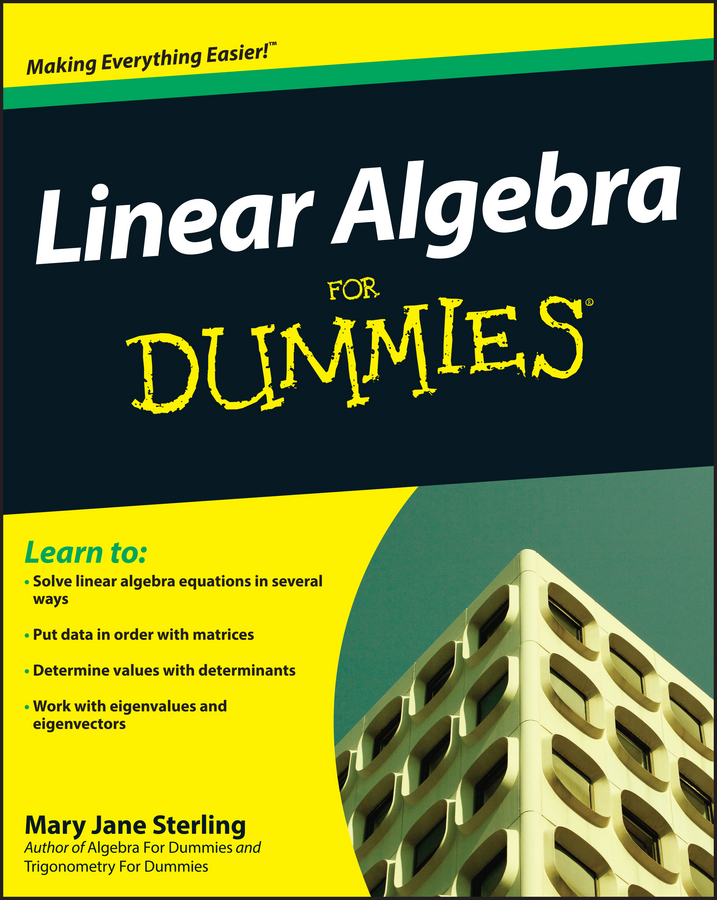##### Linear Algebra For DummiesWhether you add or subtract variables, you follow the same rule, even though they have different operations: when adding or subtracting terms that have exactly the same variables, you either add or subtract the coefficients, and let the result stand with the variable.

For example:

• Addition. In this equation, you can add all of the coefficients (2, 5, and 4) because the variables are the same (a).

2a + 5a + 4a = 11a

• Subtraction. In this equation, you can subtract all of the coefficients (11, 5, and 4) because the variables are the same (a).

11a – 5a – 4a = 2a

If no number appears before the variable, then you can assume that the number is 1. So a = 1a and x = 1x.

You add terms that have the same variables because they represent the same amounts. You don’t try to add the terms with different variables. The examples that follow involve two or more variables:

• Two variables.

a + 3a + x + 2x

(1a + 3a) + (1x + 2x)

4a + 3x

• Three variables.

5a + 2a + 6b + 8b + 11c

(5a + 2a) + (6b + 8b) + 11c

7a + 14b + 11c